How to Calculate and Solve for Standing Bubble Point Parameter | The Calculator Encyclopedia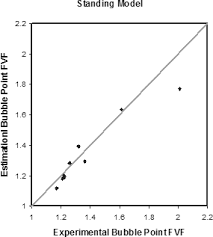The image above represents standing bubble point parameter.

To compute for the standing bubble point parameter, two essential parameters are needed and these parameters are API Gravity (°API) and Temperature (°Rankine) (T).

The formula for calculating standing bubble point parameter:

a = [0.00091(T – 460)] – [0.0125(°API)]

Where:

a = Standing Bubble Point Parameter, a
°API = API Gravity
T = Temperature (°Rankine)

Let’s solve an example;
Find the standing bubble point parameter when API Gravity is 32 and the temperature is 146.

This implies that:

°API = API Gravity = 32
T = Temperature (°Rankine) = 146

a = [0.00091(T – 460)] – [0.0125(°API)]
a = [0.00091(146 – 460)] – [0.0125(32)]
a = [0.00091(-314)] – [0.0125(32)]
a = [-0.28574] – [0.0125(32)]
a = [-0.28574] – [0.4]
a = -0.68574

Therefore, the standing bubble point parameter, a is -0.68574.

How to Calculate and Solve for Glass Gas Solubility in a Fluid | The Calculator Encyclopedia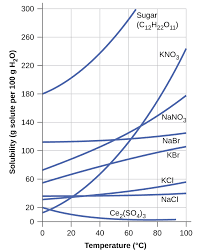The image above represents glass gas solubility.

To compute for the glass gas solubility, four essential parameters are needed and these parameters are API Gravity (°API), Temperature (°Rankine) (T), Mean Bubble Point (Pb*) and Gas Gravity at Actual Separator Psep and Tsepg).

The formula for calculating the glass gas solubility:

γgs = γg[((°API)0.989 / (T – 460)0.172)Pb*]1.2255

Where:

γgs = Glass Gas Solubility
°API = API Gravity
T = Temperature (°Rankine)
Pb* = Mean Bubble Point
γg = Gas Gravity at Actual Separator Psep and Tsep

Let’s solve an example;
Find the glass gas solubility when the API Gravity is 15, the temperature is 30, the mean bubble point is 40 and the gas gravity at actual separator is 54.

This implies that;

°API = API Gravity = 15
T = Temperature (°Rankine) = 30
Pb* = Mean Bubble Point = 40
γg = Gas Gravity at Actual Separator Psep and Tsep = 54

γgs = γg[((°API)0.989 / (T – 460)0.172)Pb*]1.2255
γgs = 54[((15)0.989 / (30 – 460)0.172)40]1.2255
γgs = 54[((15)0.989 / (-430)0.172)40]1.2255
γgs = 54[((15)0.989 / (NaN))40]1.2255
γgs = 54[(14.5 / NaN)40]1.2255
γgs = 54[(NaN)40]1.2255
γgs = 54[NaN]1.2255
γgs = 54[NaN]
γgs = NaN

Therefore, the glass gas solubility is NaN.

How to Calculate and Solve for Separator Gas Gravity in a Fluid | The Calculator Encyclopedia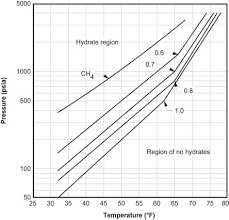The image above represents separator gas gravity.

To compute for the separator gas gravity, four essential parameters are needed and these parameters are API Gravity (°API), Actual Separator Temperature (°Rankine) (Tsep), Actual Separator Pressure (Psep) and Gas Gravity at Actual Separator Psep and Tsepg).

The formula for calculating the separator gas gravity:

γgs = γg[1 + 5.912(10-5)(°API)(Tsep – 460)log(Psep / 114.7)]

Where:

γgs = Separator Gas Gravity
°API = API Gravity
Tsep = Actual Separator Temperature (°Rankine)
Psep = Actual Separator Pressure
γg = Gas Gravity at Actual Separator Psep and Tsep

Let’s solve an example;
Find the separator gas gravity with an API Gravity of 21, actual separator temperature of 18, actual separator pressure of 14 and gas gravity at actual separator of 32.

This implies that;

°API = API Gravity = 21
Tsep = Actual Separator Temperature (°Rankine) = 18
Psep = Actual Separator Pressure = 14
γg = Gas Gravity at Actual Separator Psep and Tsep = 32

γgs = γg[1 + 5.912(10-5)(°API)(Tsep – 460)log(Psep / 114.7)]
γgs = 32[1 + 5.912(10-5)(21)(18 – 460)log(14 / 114.7)]
γgs = 32 [1 + 5.912(10-5)(21)(18 – 460) log(0.1220)]
γgs = 32 [1 + 5.912(10-5)(21)(-442) log(0.1220)]
γgs = 32 [1 + 5.912(10-5)(21) (-442) (-0.9134)]
γgs = 32 [1 + 0.501]
γgs = 32 [1.501]
γgs = 48.039

Therefore, the separator gas gravity is 48.039.

How to Calculate and Solve for Gas Formation Volume Factor (FVF) | The Calculator Encyclopedia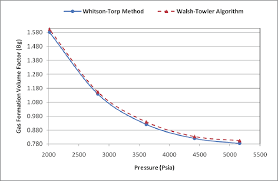The image above represents the gas FVF.

To compute for the gas FVF, three essential parameters are needed and these parameters are Z-Factor (Z), Temperature (T) and Pressure (P).

The formula for calculating the gas FVF:

Bg = 0.00504[ZT / P]

Where;

Bg = Gas FVF
Z = Z-Factor
T = (°R) Temperature
P = Pressure

Let’s solve an example;
Find the Gas FVF when the Z-Factor is 12, temperature is 30 and the pressure is 120.

This implies that;

Z = Z-Factor = 12
T = (°R) Temperature = 30
P = Pressure = 120

Bg = 0.00504[ZT / P]
Bg = 0.00504[12 x 30 / 120]
Bg = 0.00504[360 / 120]
Bg = 0.00504
Bg = 0.01512

Therefore, the gas FVF is 0.01512 bbl / scf.

Calculating for the Z-Factor when the Gas FVF, Temperature and Pressure is Given.

Z = Bg.P / 0.00504T

Where;

Z = Z-Factor
Bg = Gas FVF
T = (°R) Temperature
P = Pressure

Let’s solve an example;
Find the Z-Factor when the Gas FVF is 22, temperature is 16 and the pressure is 80.

This implies that;

Bg = Gas FVF = 22
T = (°R) Temperature = 16
P = Pressure = 80

Z = Bg.P / 0.00504T
Z = 22 x 80 / 0.00504 x 16
Z = 1760 / 0.08064
Z = 21825.39

Therefore, the Z-Factor is 21825.39.

Calculating for the Temperature when the Gas FVF, Z-Factor and the Pressure is Given.

T = Bg.P / 0.00504Z

Where;

T = (°R) Temperature
Z = Z-Factor
Bg = Gas FVF
P = Pressure

Let’s solve an example;
Find the Temperature when the Gas FVF is 44, Z-Factor is 29 and the pressure is 70.

This implies that;

Z = Z-Factor = 29
Bg = Gas FVF = 44
P = Pressure = 70

T = Bg.P / 0.00504Z
T = 44 x 70 / 0.00504 x 29
T = 3080 / 0.14616
T = 21072.79

Therefore, the temperature is 21072.79.

How to Calculate and Solve for Temperature, Number of Moles, Volume, Van’t Hoff Factor and Osmotic Pressure | The Calculator Encyclopedia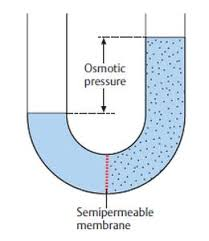The image above represents the osmotic pressure.

To compute for the osmotic pressure, five parameters are needed and these parameters are Ideal Gas Constant (R)Temperature in Kelvin (T), Number of Moles (n), Volume (V) and Van’t Hoff’s Factor (i).

The formula for calculating osmotic pressure:

π = i nRTV

Where;
π = osmotic pressure
n = number of moles
R = ideal gas constant
T = temperature in Kelvin
i = Van’t Hoff’s Factor
V = Volume

Let’s solve an example;
Find the osmotic pressure when the ideal gas constant is 0.08206 with a temperature in kelvin of 120, number of moles is 32, a volume of 48 and a van’t hoff’s factor of 24.

This implies that;
n = number of moles = 32
R = ideal gas constant = 0.08206
T = temperature in Kelvin = 120
i = Van’t Hoff’s Factor = 24
V = Volume = 48

π = i nRTV
π = 24 32 x 0.08206 x 12048
π = (24) (315.110)(48)
π = (24)(6.5647)
π = 157.5

Therefore, the osmotic pressure is 157.5 atm.

Calculating the Van’t Hoff’s Factor using the Osmotic Pressure, Number of Moles, Temperature in Kelvin, Ideal Gas Constant and Volume.

i = / nRT

Where;
i = Van’t Hoff’s Factor
π = osmotic pressure
V = Volume
n = number of moles
R = ideal gas constant
T = temperature in Kelvin

Let’s solve an example;
Find the Van’t Hoff’s Factor when the osmotic pressure is 220, volume of 50, temperature in kelvin of 180 and number of moles of 60. (R = 0.08206)

This implies that;
π = osmotic pressure = 220
V = Volume = 50
n = number of moles = 60
R = ideal gas constant = 0.08206
T = temperature in Kelvin = 180

i = / nRT
i = 50 x 220 / 60 x 0.08206 x 180
i = 11000 / 866.808
i = 12.69

Therefore, the Van’t Hoff’s Factor is 12.69.

Calculating the Volume using the Osmotic Pressure, Number of Moles, Temperature in Kelvin, Ideal Gas Constant and Van’t Hoff’s Factor.

V = i (nRT) / π

Where;
V = Volume
i = Van’t Hoff’s Factor
π = osmotic pressure
n = number of moles
R = ideal gas constant
T = temperature in Kelvin

Let’s solve an example;
Find the volume when the osmotic pressure is 280, Van’t Hoff’s Factor of 40, temperature in kelvin of 90 and number of moles of 70. (R = 0.08206)

This implies that;
i = Van’t Hoff’s Factor = 40
π = osmotic pressure = 280
n = number of moles = 70
R = ideal gas constant = 0.08206
T = temperature in Kelvin = 90

V = i (nRT) / π
V = 40 (70 x 0.08206 x 90) / 280
V = 40 (516.978) / 280
V = 20679.12 / 280
V = 73.854

Therefore, the volume is 73.854.

Nickzom Calculator Solves Calculations on Gas Laws

In Physics and Chemistry, there are four prominent gas laws that govern calculations related to gases. These laws have major parameters such as:

• Pressure
• Temperature
• Volume

The four gas laws are:

• Boyle’s Law
• Charles’ Law
• Pressure or Gay Lussac’s Law
• The General Gas Equation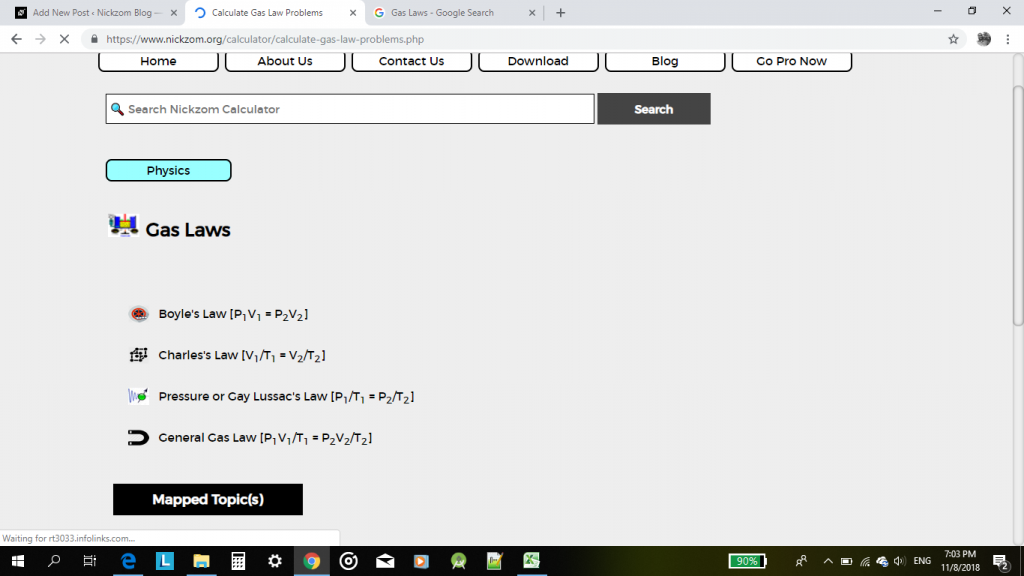According to Boyle’s law, pressure is indirectly proportional to volume.

According to Charles’ law, volume is directly proportional to temperature.

According to Pressure or Gay Lussac’s law, pressure is directly proportional to temperature.

According to the General Gas Equation or law, the product of pressure and volume is directly proportional to temperature.

For this post, I would be given an example of how Nickzom Calculator can solve a problem on gas laws with the general gas equation or law.

One can access Nickzom Calculator via any of these channels:

Let’s assume that one is looking for an unknown temperature.

First and foremost, click on General Gas Law.

Then click on Unknwon Temperature.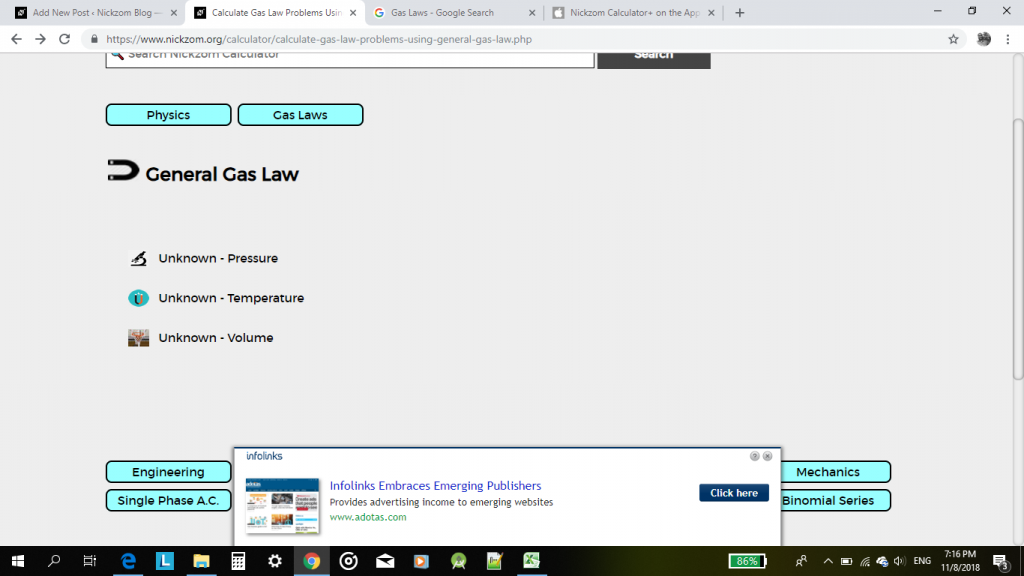Now, proceed to enter the values of the known parameters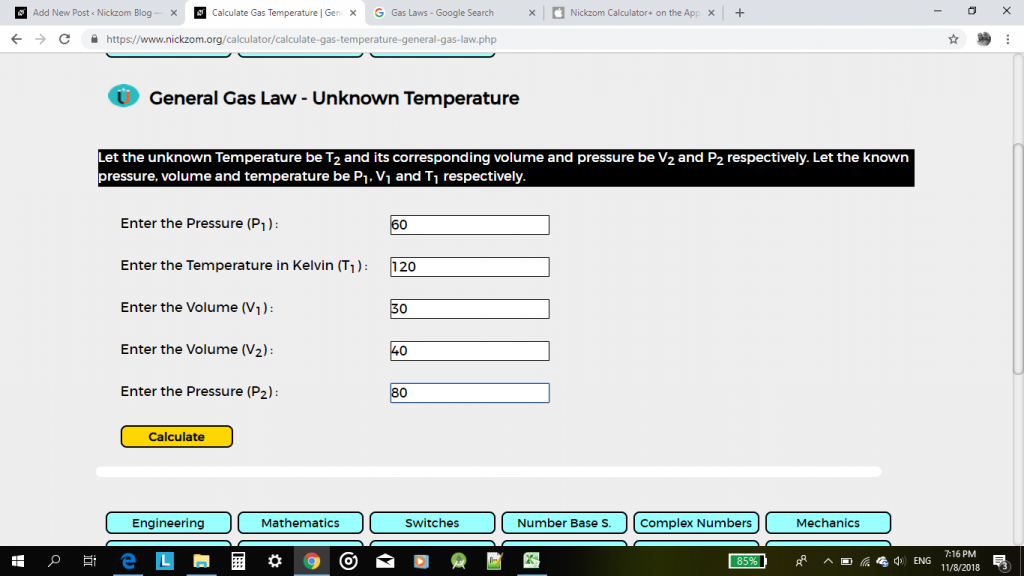Then, click on calculate.

The result would be displayed for you in less than a second.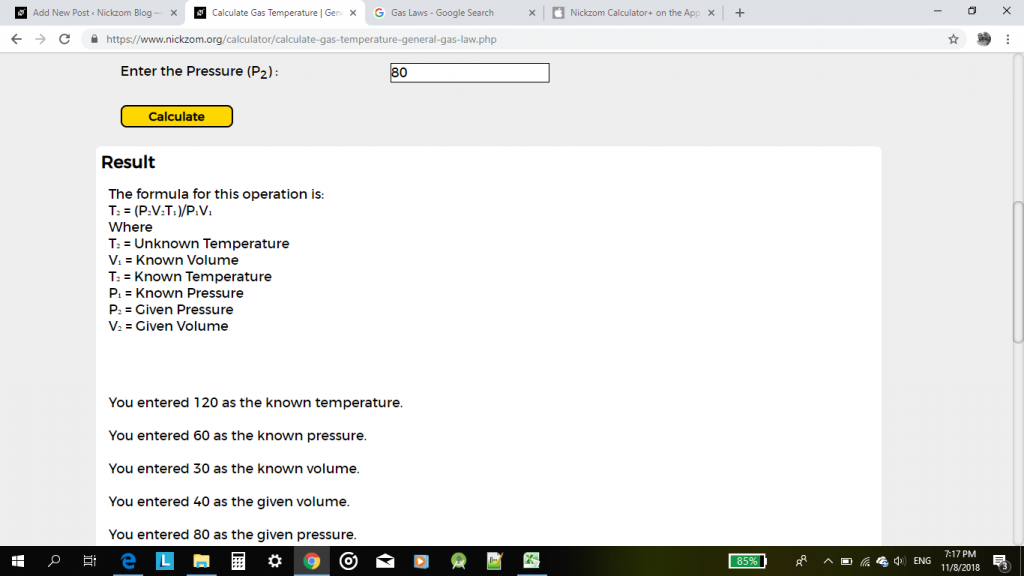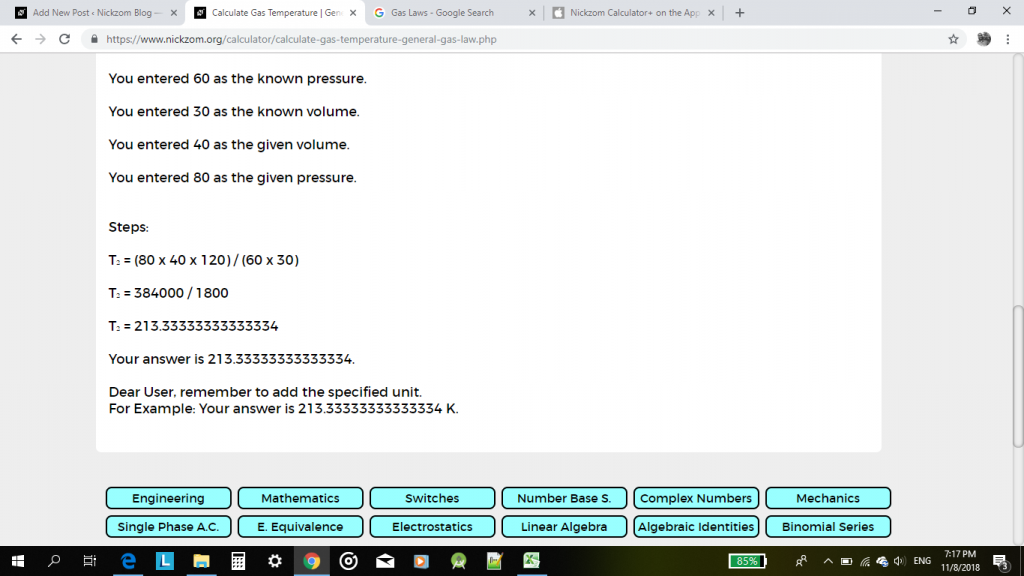This pattern can be used to solve any calculation problem one faces in gas laws.